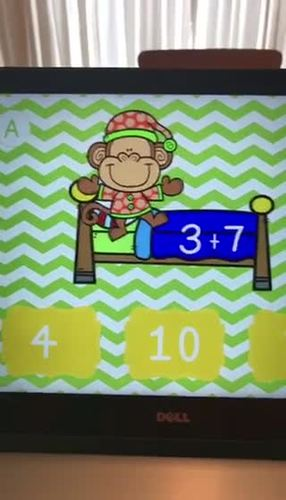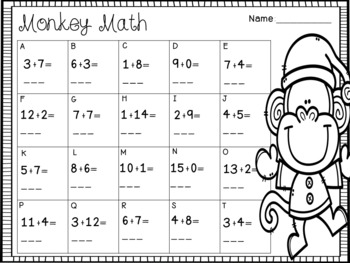# Monkey Math Addition Interactive PPTResource Type
File Type

Zip

(11 MB|22 pages)
Product Rating
Standards
• Product Description
• StandardsNEW
Please enjoy this interactive power point in your classroom as a way to strengthen addition fluency. This can be used whole group, small group or with individual students.

There are 20 addition facts with sums to 15. Each slide has 3 possible answers. When the correct sum is selected, the monkey will dance on the bed. Students can record their answer then click the arrow to advance to the next slide.
Add and subtract within 20, demonstrating fluency for addition and subtraction within 10. Use strategies such as counting on; making ten (e.g., 8 + 6 = 8 + 2 + 4 = 10 + 4 = 14); decomposing a number leading to a ten (e.g., 13 - 4 = 13 - 3 - 1 = 10 - 1 = 9); using the relationship between addition and subtraction (e.g., knowing that 8 + 4 = 12, one knows 12 - 8 = 4); and creating equivalent but easier or known sums (e.g., adding 6 + 7 by creating the known equivalent 6 + 6 + 1 = 12 + 1 = 13).
Fluently add and subtract within 5.
Total Pages
22 pages
N/A
Teaching Duration
N/A
Report this Resource to TpT
Reported resources will be reviewed by our team. Report this resource to let us know if this resource violates TpT’s content guidelines.